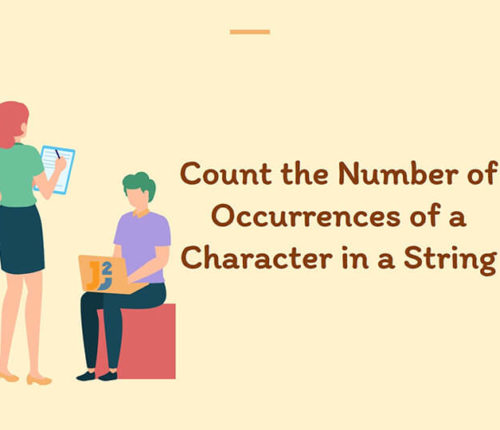•05 April

### Count occurrences of Character in String in Java

In this article, we will look at a problem: Given an Input String and a Character, we have to Count Occurrences Of character in String in java. For Example, If the Given String is : "Java2Blog" and we have to count the occurrences of Character ‘a’ in the String. Therefore, Count of 'a' is : […]

•24 December

### How to capitalize first letter in java

Learn about how to capitalize first letter in java.

•09 October

### Java program to find first and last digit of a number

In this article, we are going to find first and last digit of a number in Java. To find first and last digit of any number, we can have several ways like using modulo operator or pow() and log() methods of Math class etc. Let’s see some examples. Before moving to example, let’s first create […]

•30 September

### Find Perfect Number in Java

In this article, we are going to find whether a number is perfect or not using Java. A number is called a perfect number if the sum of its divisors is equal to the number. The sum of divisors excludes the number. There may be several approaches to find the perfect number. For example: 28 […]

•28 September

### Number guessing game in java

In this article, we will implement Number guessing game in java. The number guessing game is based on a concept where player guesses a number between a range. If player guesses the exact number then player wins else player looses the game. Since this game provides limited attempts, so, player must guess the number with […]

•03 October

### Happy Number program in Java

In this article, we are going to learn to find Happy Number using Java. Let’s first understand, what is Happy Number? What is a Happy Number? A number which leaves 1 as a result after a sequence of steps and in each step number is replaced by the sum of squares of its digit. For […]

•28 September

### How to find Magic Number in Java

In this article, we are going to learn to find Magic Number using Java. Let’s first understand, what is Magic Number? What is a Magic Number? A number which leaves 1 as a result after a sequence of steps and in each step number is replaced by the sum of its digits. For example, if […]

•18 September

### Return second last digit of given number in java

In this topic, we will learn to get the second last digit of a number in Java with the help of examples. There can be several ways to get second last digit; here we are discussing some solutions. Using modulo operator Here, we are using modulo and divide operators to find the second last digit. […]

•05 October

### Calculate total surface area of Hemisphere in java

In this post, we will see how to calculate total surface area of Hemisphere in java. Hemisphere is exactly half of sphere. There can be many practical examples of Hemisphere. You can divide earth into two hemisphere i.e. Northen Hemisphere and Southern Hemisphere. Formula of calculating total surface area of Hemisphere is: surface area of […]